×#### Thank you for registering.

One of our academic counsellors will contact you within 1 working day.

Click to Chat

1800-1023-196

+91-120-4616500

CART 0

• 0

MY CART (5)

Use Coupon: CART20 and get 20% off on all online Study Material

ITEM
DETAILS
MRP
DISCOUNT
FINAL PRICE
Total Price: Rs.

There are no items in this cart.
Continue Shopping• Complete JEE Main/Advanced Course and Test Series
• OFFERED PRICE: Rs. 15,900
• View Details

```Chapter 3: Pair of Linear Equations in Two Variables Exercise – 3.1

Question: 1

Akhila went to a fair in her village. She wanted to enjoy rides on the giant wheel and play hoopla (a game in which you throw a rig on the items in the stall, and if the ring covers any object completely you get it). The number of times she played hoopla is half the number of rides she played she had on a the giant wheel. Each ride costs Rs.3 and a game of hoopla costs Rs.4. If she spent Rs.20 in the fair, represent this situation algebraically and graphically.

Solution:

The pair of equation formed is:x – 2y = 0                            ………(i)

3x + 4y = 20                       ……… (ii)

Let us represent these equations graphically. For this, we need at least two Solutions for each Q. We give these Solutions in the table:

x
0
20
1

x
0
2
4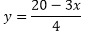5
0
2

When: The solution of the variable is zero; the equation can be solved easily. Putting x = 0 in equation (ii) we get

4y = 20

i.e. y = 5

Similarly putting y = 0 in equation (ii) we get

3x = 20

x = 20/3 but it is not an integer so it is not easy to plot on graph paper so we chose y = 2 which gives x = 4 as an integer value.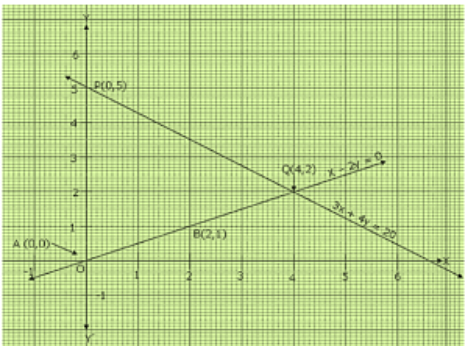The points are A(0, 0); B(0, 5) and P(0, 5) ; Q(4, 12)

Question: 2

Aftab tells his daughter, ”Seven years ago, I was seven times as old as you were then. Also, three years from now, I shall be three times as old as you will be.” Is this not interesting? Represent this situation algebraically and graphically

Solution:

Let the present age of Aftab and his daughter be x and y respectively. Seven years ago,

Age of Aftab = x – 7

Age of his daughter = y – 7

According to the given condition,

x – 7 = 7

(y – 7) = x – 7y = -42

Three years from hence,

x + 3 = 3

(y + 3) = x – 3y = 6

Thus the given Sol: can be algebraically represented as

= x – 7y = – 42          ……….. (i)

= x – 3y = 6              ……… (ii)

From equation (i)

x = – 42 + 7y

x
-7
0
7

y
5
6
7

From equation (ii)

x = 6 + 3y

x
6
3
0

y
0
-1
-2

The above can be plotted in the graph as follows: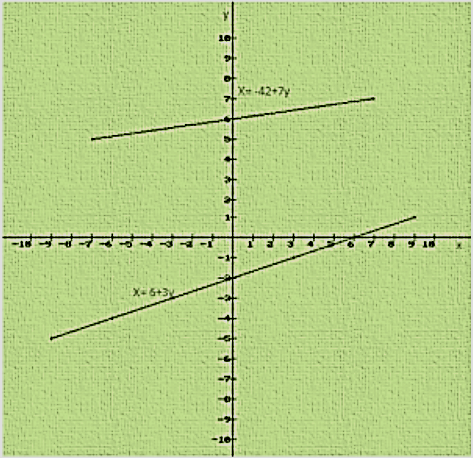Question: 3

The path of the train A is given by the equation 3x + 4y - 12 = 0 and the path of another train B is given by the equation 6x + 8y - 48 = 0. Represent this situation graphically.

Solution:

The paths of two trains are given by the following pair of linear equations.

3x + 4y – 12 = 0          ………..(i)

6x + 8y – 48 = 0          ………..(ii)

In order to represent the following sets of lines graphically we need two points for a single equation

We have,

3x + 4y – 12 = 0

Putting y = 0

3x + 4(0) = 12 = 3x = 12 = x = 4

Hence the coordinate is (4, 0)

Putting x = 0

3(0) + 4y = 12 = 4y = 12 = y = 3

Hence the coordinate is (0, 3)

We have,

6x + 8y – 48 = 0

Putting x = 0

= 6(0) + 8y = 48 = 8y = 48 = y = 6

Hence the coordinate is (0, 6)

6x + 8y – 48 = 0

Putting y = 0

6x + 8(0) = 48 = 6x = 48 = x = 8

Hence the coordinate is (8, 0)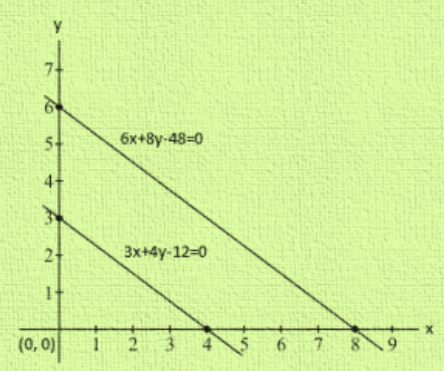Hence the two lines intersect at point (-1, 2)

Hence x = -1 and y = 2

Question: 4

Gloria is walking along a path joining (-2, 3) and (2,- 2), while Suresh is walking along the path joining (0, 5) and (4, 0). Represent this situation graphically.

Solution:

It is given that Gloria is walking along the path joining (-2, 3) and (2,- 2), while Suresh is walking along the path joining (0, 5) and (4, 0)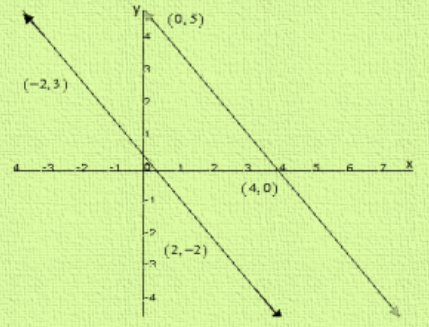We observed that the two lines did not intersect anywhere, hence they are parallel.

Question: 5

On comparing the ratiosand without drawing them, find out whether the lines representing the following pairs of linear equations intersect at a point, are parallel or co-incide:

(i) 5x - 4y + 8 = 0 and 7x + 6y - 9 = 0

(ii) 9x + 3y +12 = 0 and 18x + 6y + 24 = 0.

(iii) 6x - 3y +10 = 0 and 2x - y + 9 = 0

Solution:

(i) 5x - 4y + 8 = 0 and 7x + 6y – 9 = 0

Here a1 = 5, b1 = – 4, c1 = 8 = a2 = 7, b2 = 6, c2 = – 9

We have,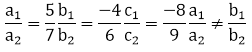Two lines are intersecting at a point

(ii) 9x + 3y + 12 = 0 and 18x + 6y + 24 = 0.

Here a1= 9, b1= 3 ,c1= 12 = a2 = 18, b2 = 6,c2 = 24

We have,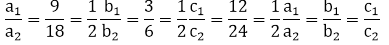Both the lines co –inside with each other.

(iii) 6x - 3y +10 = 0 and 2x - y + 9 = 0

Here a1 = 6, b1 = -3, c1 = 10 = a2 = 2, b2 = -1, c2 = 9

We have,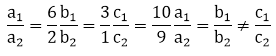Therefore the lines are parallel.

Question: 6

Given the linear equation 2x + 3y – 8 = 0, write another linear equation in two variable such that the geometrical representation of the pair so formed are.

(i) Intersecting lines

(ii) Parallel lines

(iii) Coincident lines

Solution:

We have, 2x + 3y - 8 = 0

Let another equation of line is 4x + 9y - 4 = 0

Here, Here a1 = 2, b1 = 3, c1 = – 8

= a2 = 4, b2 = 9, c2 = - 4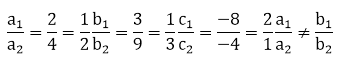2x + 3y - 8 = 0 and 4x + 9y - 4 = 0 intersect each other at a point.

Hence, the required equation of the line is 4x + 9y - 4 = 0

We have, 2x + 3y - 8 = 0

Let another equation of line is 4x + 6y - 4 = 0

Here, Here a1 = 2, b1 = 3, c1 = – 8

= a2 = 4, b2 = 6, c2 = – 4Therefore the lines are parallel to each other.

Hence, the required equation of the line is 4x + 6y- 4 = 0

Question: 7

The cost of 2kg apples and 1kg of grapes was found to be Rs.160.After a month the cost of 4 kg of apples and 2 kg of grapes is Rs.300. represent this situation algebraically and geometrically.

Solution:

Let the cost of a 1kg apple and 1 kg grape be Rs x and Rs. y respectively.

The given conditions can be algebraically represented as:

2x + y = 160        ………… (i)

4x + 2y = 300     ………… (ii)

From equation (i)

Y =  160 – 2x

x
50
60
70

y
60
40
20

From equation (ii):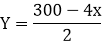x
70
80
75

y
10
-10
0

The graphical representation is as follows: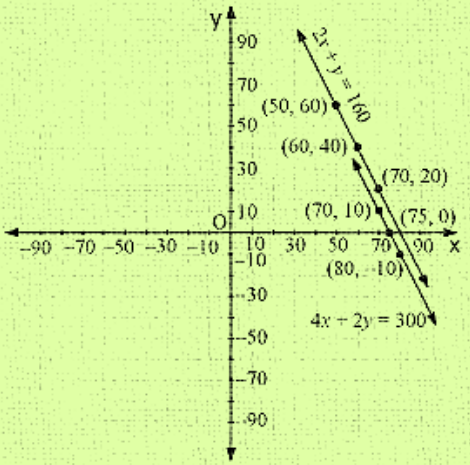```### Course Features

• 728 Video Lectures
• Revision Notes
• Previous Year Papers
• Mind Map
• Study Planner
• NCERT Solutions
• Discussion Forum
• Test paper with Video Solution